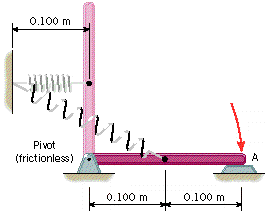# Harmonic Motion

A 0.200 m uniform bar has a mass of 0.770 kg and is released from rest in the vertical position, as the drawing indicates. The spring is initially unstrained and has a spring constant of k = 28.0 N/m. Find the tangential speed with which end A strikes the horizontal surface.---

I've been struggling with this problems for quite a while now.
I know that:
$$x = \theta r$$

$$v _{f} = \frac{x}{t}$$

Beyond these two simple steps I can't figure anything out... i tried experimenting by integrating with respect to theta and x, but it still didnt work out... any help would be great. Thanks.

Last edited:

wlvanbiesen,

The first way I thought of was to consider the torques (there are two of them) that act on the rod. The rod has a moment of inertia about its pivot point, so you'd integrate angular acceleration over the time it takes for the rod to go from vertical to horizontal....That looked like a mess!

But then I thought of energy conservation! Give that a try; I think it will work.

krab
I would use energy. You know the potential gravitational energy before and after and the potential spring energy after. The left over energy is the kineti energy (1/2)I omega^2, where I is the moment of inertia of the bar.